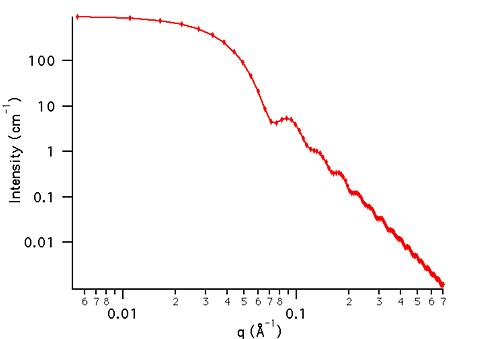MODEL

PolyRectSphere

AUTHOR/MODIFICATION

Steve Kline 20 JAN 1999

Alan Munter 08 JULY 1999, converted to Java

APPROVED FOR DISTRIBUTION

DESCRIPTION

Calculates the form factor for a polydisperse population of spheres with uniform scattering length density. The distribution of radii is a rectangular (box) distribution. The form factor is normalized by the average particle volume such that P(q) = scale*<f*f>/Vol + bkg, where f is the single particle scattering amplitude and the < > denote an average over the size distribution.

Resolution smeared version is also provided.

VARIABLES

Input Variables (default values):

Parameter Variable Value
0Scale1.0
2Polydispersity (0-1)0.12
3Contrast (Å-2)3.0e-6
4Incoherent Background(cm-1)0.000

USAGE NOTES

The returned value is scaled to units of [cm-1], absolute scale.

contrast = SLD (sphere) - SLD (solvent)

The (normalized) rectangular distribution is: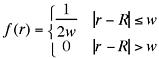with the constraint that w <= R. Here R is the average radius specified by parameter above.

R is the mean of the distribution and w is the half-width. The root mean square deviation is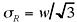. The polydispersity,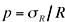.

The form factor is normalized by the average volume, using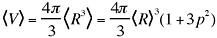.

If the scale factor Parameter is set equal to the particle volume fraction, phi, the returned value is the scattered intensity per unit volume, I(q) = phi*P(q). However, no interparticle interference effects are included in this calculation.

Parameter (scale) and Parameter (contrast) are multiplicative factors in the model and are perfectly correlated. Only one of these parameters should be left free during model fitting.

REFERENCE

Kotlarchyk, M.; Chen, S.-H. J. Chem. Phys., 1983, 79, 2461.

TEST DATASET

This example dataset is produced by calculating the PolyRectSphere using 128 data points, qmin = 0.001 Å-1, qmax = 0.7 Å-1 and the above default parameter values.